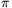Mathematical and Physical Journal
for High Schools
Issued by the MATFUND Foundation
 Already signed up? New to KöMaL?

#Problem A. 428. (May 2007)

A. 428. Given a convex lattice polygon such that both x- and y-coordinates of each vertex lie in the interval [0,n]. Show that the number of vertices is less than 100n2/3.

(5 pont)

Deadline expired on June 15, 2007.

Solution. The number of vertices is trivially at most 4n, so we can assume that n56 and the number of vertices is at least 54.

Let the side vectors of the polygon be (in counterclockwise order) v1,v2,...,vk, where k54 is the number of vertices. Since the perimeter of the convex polygon cannot be greater than the perimeter of the square containing it,(1)

Sort the integer vectors in the plane by their lengths:, ahol 0=|u0|<|u1||u2||u3|....

For an arbitrary positive integer m, consider the vectors u0,...,um. Drawing a unit square around their end-points, these squares do not overlap and they are contained in a circle of radius |un|+1. Therefore m+1<(|vm|+1)2, andSubstituting into (1),Hence,

k<(16n)2/3<100n2/3.

### Statistics:

 8 students sent a solution. 5 points: Gyenizse Gergő, Kónya 495 Gábor, Lovász László Miklós, Nagy 224 Csaba, Nagy 235 János, Nagy 314 Dániel, Tomon István. Unfair, not evaluated: 1 solutions.

Problems in Mathematics of KöMaL, May 2007